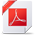# SPSS Statistics

View Only

## Calculation of CI-bounds for a poisson model with offset-parameter#### Maike BestehornMon September 13, 2021 11:24 AM• #### 1.  Calculation of CI-bounds for a poisson model with offset-parameter

Posted Mon September 13, 2021 11:24 AM
Hello,
I am struggling with CI-calculation for a GENLIN-Model.
I calculated a poisson-model with offset-variable and saved the lower and upper CI-bounds for the mean of the response. These values for the CI-bounds are different to the calculation with the values of the parameter estimation table (see attachement)
Where can I find the documentation how the CI-values are calculated?

------------------------------
Maike Bestehorn
------------------------------

Attachment(s)• #### 2.  RE: Calculation of CI-bounds for a poisson model with offset-parameter

Posted Mon September 13, 2021 11:52 AM